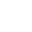# PHP Operators

In all programming languages, operators are used to manipulate or perform operations on variables and values. You have already seen the string concatenation operator “.” in theÂ Echo LessonÂ and the assignment operator “=” in pretty much every PHP example so far.

There are many operators used in PHP Â i.e. PHP Operators, so we have separated them into the following categories to make it easier to learn them all.

• Assignment Operators
• Arithmetic Operators
• Comparison Operators
• String Operators
• Combination Arithmetic & Assignment Operators

# assignment operators

Assignment operators are used to set a variable equal to a value or set a variable to another variable’s value. Such an assignment of value is done with the “=”, or equal character. Example:

Now both \$my_var and \$another_var contain the value 4. Assignments can also be used in conjunction with arithmetic operators.

# 1. Arithmetic operators

These operators are PHP Operators used to perform arithmetic operations like in algebra. There are different arithmetic operations, so there are that many type of arithmetic operators as well in PHP so that all operations can be performed easily. These are:

• ### Modulus ( % )

We donâ€™t think we need to explain whatâ€™s these operators and how these works. We will give one example for each which will explain the purpose and use of each operator.

# Modulus ( % )

All the above four operators don’t need explanation. But here with this operators, we need a little explanation that what this operator is and where we can use this operator.

This operator gives the remainder of the division operation.

# 2. Comparison operators

Comparisons are used to check the relationship between variables and/or values.

Comparison operators are used inside conditional statements and evaluate to eitherÂ trueÂ orÂ false. Here are the most important comparison PHP operators.
Assume: \$x = 4 and \$y = 5;

 Operator English Example Result == Equal To \$x == \$y false != Not Equal To \$x != \$y true < Less Than \$x < \$y true > Greater Than \$x > \$y false <= Less Than or Equal To \$x <= \$y true >= Greater Than or Equal To \$x >= \$y false

# 3. Combination arithmetic & assignment operators

In programming it is a very common task to have to increment a variable by some fixed amount. The most common example of this is a counter. Say you want to increment a counter by 1, you would have:

\$counter = \$counter + 1;

However, there is a shorthand for doing this.

\$counter += 1;

This combination assignment/arithmetic operator would accomplish the same task. The downside to this combination operator is that it reduces code readability to those programmers who are not used to such an operator. Here are some examples of other common shorthand operators. In general, “+=” and “-=” are the most widely used combination operators.

 Operator English Example Equivalent Operation += Plus Equals \$x += 2; \$x = \$x + 2; -= Minus Equals \$x -= 4; \$x = \$x – 4; *= Multiply Equals \$x *= 3; \$x = \$x * 3; /= Divide Equals \$x /= 2; \$x = \$x / 2; %= Modulo Equals \$x %= 5; \$x = \$x % 5; .= Concatenate Equals \$my_str.=”hello”; \$my_str = \$my_str . “hello”;

# 4.Â pre/post-increment & pre/post-decrement

This may seem a bit absurd, but there is even a shorter shorthand for the common task of adding 1 or subtracting 1 from a variable. To add one to a variable or “increment” use the “++” operator:

\$x++; Which is equivalent to \$x += 1; or \$x = \$x + 1;

To subtract 1 from a variable, or “decrement” use the “–” operator:

\$x–; Which is equivalent to \$x -= 1; or \$x = \$x – 1;

In addition to this “shorterhand” technique, you can specify whether you want to increment before the line of code is being executed or after the line has executed. Our PHP code below will display the difference.

This is the end of the Chapter PHP Operators.Automated page speed optimizations for fast site performance